# Investigating Unexpected Results in Elementary Experiment

Gold Member
Homework Statement
find experimentally and theoretically the horizontal distance a slingshot which roughly obeys Hooke's Law will shoot a projectile.
Relevant Equations
F=kx (x = distance stretched), range = v*(sin(2A))/g, (v = velocity upon leaving slingshot, A = angle to horizontal of v, g = acceleration due to gravity); v = x*sqrt(2k/m) , (m=mass of projectile,)
This concerns an elementary experiment that I (a teacher) have done with several secondary school classes, up until now with success. However, I gave the same instructions to a homeschooling student (in another country , so I couldn’t actually directly oversee the experiment), and the experiment’s results were off by a factor of two to the predictions by the tried-and-true calculations. Naturally it is difficult to check something like this from afar, but perhaps someone can suggest reasons for which the experiment was so far off.

The experiment makes a large simplification, which admittedly is strictly not valid but has always served as a decent approximation. The assumption is that the rubber strip in a slingshot roughly obeys Hooke’s Law.

The experiment is then

(a) find a value for k in Hooke’s Law F=kx experimentally by hanging several weights to it (but careful not to use weights which were too heavy) versus the length this stretches the rubber band (including the length of stretch that one will use to shoot) to shoot a projectile . From the straight-line graph (force versus stretch distance) one obtains the spring constant.

(b) Measure the angle A to the horizontal that one will shoot the projectile of mass m, and the length x' that one will stretch the rubber band in the same way as was done in (a).

(c) shoot the projectile (several times) , and measure the horizontal distance D (from the slingshot) that it lands.

(d) calculate the theoretical horizontal distance D’ (in steps, rather than a single formula, but that is irrelevant) from the measured k, x', m and A.

Usually D and D’ are pretty close, despite ignoring friction, heat loss, and other minor influences. (I did not see any undue friction in her experimental set-up.)

I checked the student’s calculations, and they were correct.

I also see no problem in measuring the restoring force in a vertical position although the shooting takes place at an angle.

The projectile’s weight of 23 grams seemed to me to be appropriate.

The most likely candidate for a problem is in the value of k. The student’s graph for k indicates that the rubber strip is following Hooke’s Law quite closely. I did have her calculate k a second time after performing the experiment, and indeed it had changed quite a bit (rubber stretches), but even using this new, lower k her calculated D’ was twice the value of her measured D.

My first instinct is to have her measure/calculate k several times to see what sort of variability k might have for the rubber strip.

However, if that doesn’t help --- what am I missing?

Thanks for any suggestions.

Homework Statement:: find experimentally and theoretically the horizontal distance a slingshot which roughly obeys Hooke's Law will shoot a projectile.
Relevant Equations:: F=kx (x = distance stretched), range = v*(sin(2A))/g, (v = velocity upon leaving slingshot, A = angle to horizontal of v, g = acceleration due to gravity); v = x*sqrt(2k/m) , (m=mass of projectile,)

This concerns an elementary experiment that I (a teacher) have done with several secondary school classes, up until now with success. However, I gave the same instructions to a homeschooling student (in another country , so I couldn’t actually directly oversee the experiment), and the experiment’s results were off by a factor of two to the predictions by the tried-and-true calculations. Naturally it is difficult to check something like this from afar, but perhaps someone can suggest reasons for which the experiment was so far off.

The experiment makes a large simplification, which admittedly is strictly not valid but has always served as a decent approximation. The assumption is that the rubber strip in a slingshot roughly obeys Hooke’s Law.

The experiment is then

(a) find a value for k in Hooke’s Law F=kx experimentally by hanging several weights to it (but careful not to use weights which were too heavy) versus the length this stretches the rubber band (including the length of stretch that one will use to shoot) to shoot a projectile . From the straight-line graph (force versus stretch distance) one obtains the spring constant.

(b) Measure the angle A to the horizontal that one will shoot the projectile of mass m, and the length x' that one will stretch the rubber band in the same way as was done in (a).

(c) shoot the projectile (several times) , and measure the horizontal distance D (from the slingshot) that it lands.

(d) calculate the theoretical horizontal distance D’ (in steps, rather than a single formula, but that is irrelevant) from the measured k, x', m and A.

Usually D and D’ are pretty close, despite ignoring friction, heat loss, and other minor influences. (I did not see any undue friction in her experimental set-up.)

I checked the student’s calculations, and they were correct.

I also see no problem in measuring the restoring force in a vertical position although the shooting takes place at an angle.

The projectile’s weight of 23 grams seemed to me to be appropriate.

The most likely candidate for a problem is in the value of k. The student’s graph for k indicates that the rubber strip is following Hooke’s Law quite closely. I did have her calculate k a second time after performing the experiment, and indeed it had changed quite a bit (rubber stretches), but even using this new, lower k her calculated D’ was twice the value of her measured D.

My first instinct is to have her measure/calculate k several times to see what sort of variability k might have for the rubber strip.

However, if that doesn’t help --- what am I missing?

Thanks for any suggestions.
Can you share the students calculations?

How are they calculating the initial velocity of the projectile? To me it seems like it's going to be an involved (to say the least) computation considering sling shot/rubber band geometry)?

Last edited:
••Thanks, erobz. Her calculations are below (if the handwriting is hard to read, I could type them out; she decided to use cgs units). She is only 13 years old, so the theory is simpler at this point (although way ahead of others her age), so of course there will lots of discrepancies from a very rigorous treatment, but I am looking for discrepancies which are huge. The construction of the slingshot is a thick rubber band attached at two points, loosely, to a board, but she shoots the projectile so that it does not make contact with the board. The k that is at the top of the calculation in blue is from data and a graph that I am not including. So, here it is: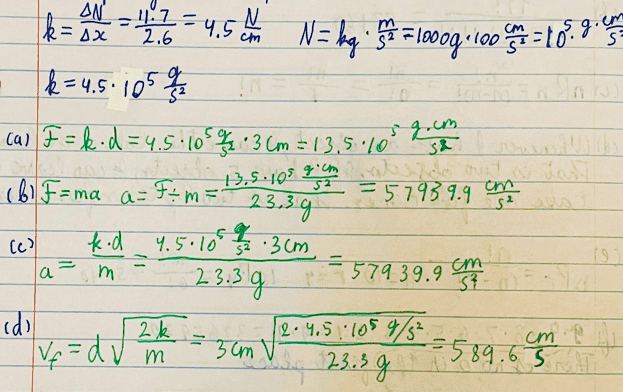•erobz
Was it this sort of slingshot? https://image.dhgate.com/0x0s/f2-al...ooden-style-slingshot-fun-traditional-kid.jpg

Is it possible that there is some sort of confusion between the length of rubber used in the calculation and the length actually used in the experiment?
Thanks, Steve4Physics. The slingshot was essentially the same as the one in your link,, but home-made and a bit clumsier for target shooting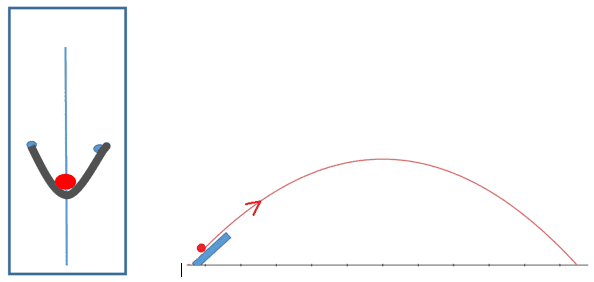The idea of a mix-up in the distances was an excellent suggestion, but I checked her calculations and found no such mix-up.

Thanks also to Mentor Berkeman for the edit.

Thanks, erobz. Her calculations are below (if the handwriting is hard to read, I could type them out; she decided to use cgs units). She is only 13 years old, so the theory is simpler at this point (although way ahead of others her age), so of course there will lots of discrepancies from a very rigorous treatment, but I am looking for discrepancies which are huge. The construction of the slingshot is a thick rubber band attached at two points, loosely, to a board, but she shoots the projectile so that it does not make contact with the board. The k that is at the top of the calculation in blue is from data and a graph that I am not including. So, here it is: View attachment 320756
The model I'm coming up with is a absolute bear.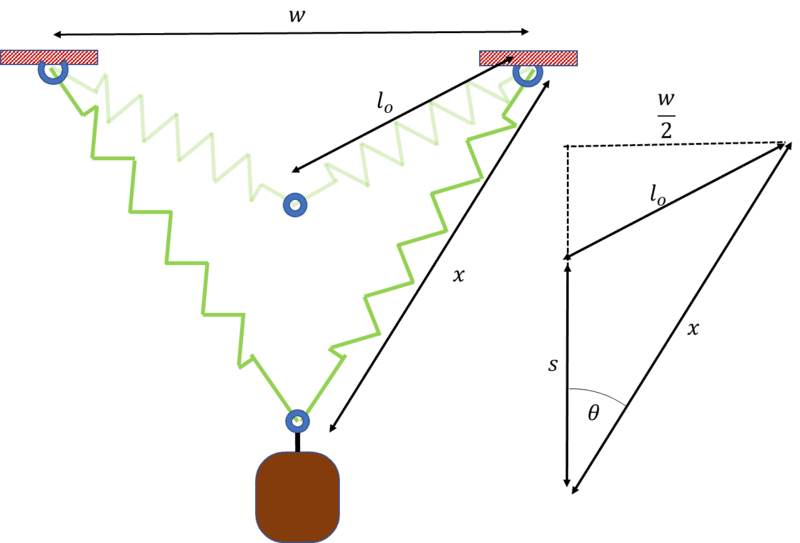$$F_{spring_{\parallel}} =2k \cos \theta ( x - l_o) = 2 k \frac{ \sqrt{ x^2 - \left( \frac{w}{2} \right)^2} }{x} ( x- l_o)$$

The work would be:

$$\frac{1}{2}m v^2 = W_{spring} = \int 2 k \frac{ \sqrt{ x^2 - \left( \frac{w}{2} \right)^2} }{x} ( x- l_o) ds$$

Where ##s## is given by the law of cosines:

$$l_o^2 = x^2 + s^2 - 2 xs \cos \theta = x^2 + s^2 - 2 s \sqrt{ x^2 - \left( \frac{w}{2} \right)^2 }$$

just getting ##\frac{ds}{dx}## is a chore, let alone integrating what follows.
Edit: In retrospect ##s## is found more readily by considering the two right triangles, rather than law of cosines.

It's interesting that turns out to be extremely reducible and give consistent results experimentally with differing material, slingshots, materials etc...

Last edited:
•Anyhow, the multiple bands (or doubled up bands) act like springs in parallel. Noticed I used ##k_{eff} = 2k##, I would use ## k_{eff} = 4k## for the sling shot shown in @Steve4Physics picture.

If you are assuming constant force, the constant force it is. Going with that, this is where my thoughts ( slingshot/band construction/geometry ) on possible error are currently residing because of post #7.

Perhaps have her carefully replicate what you are using in the classroom sling shot, or simply ship one of those sling shots to her directly.

Last edited:
•It seems to me ran (d) is wrong. There shouldn’t be a 2 under the radical .

•It seems to me ran (d) is wrong. There shouldn’t be a 2 under the radical .
I think there should be.

$$v^2 = \cancel{v_o^2}^0 + 2 a d = 2 \frac{kd}{m} d \implies v = d \sqrt{ \frac{2k}{m} }$$

Last edited:
•I think there should be.

$$v^2 = \cancel{v_o^2}^0 + 2 a d = 2 \frac{kd}{m} d \implies v = d \sqrt{ \frac{k}{m} }$$
a is not constant. The final KE must be equal to the initial stored elastic energy.

•nomadreid, MatinSAR, nasu and 2 others
a is not constant. The final KE must be equal to the initial stored elastic energy.
Yeah, I see what you are saying now. A better theory than the one that is being used is:

$$\frac{1}{2} m v^2 = \frac{1}{2} k d^2$$

But they haven't been using that theory in the classroom. They have been operating under the assumption of constant acceleration.

The issue is not the theory (and how it is being "massaged" IMHOP), it's with the repeatability of results. They are saying there is a relative error between experimental results using the same theory. What has changed is the construction of the slingshot in the case of the remote student. I think post #7 highlights why that might be an issue?

•Yeah, I see what you are saying now. A better theory than the one that is being used is:

$$\frac{1}{2} m v^2 = \frac{1}{2} k d^2$$

But they haven't been using that theory in the classroom. They have been operating under the assumption of constant acceleration.
I don't see anything in what @nomadreid wrote to suggest what method was used to determine the launch velocity. I only see that the student in question assumed constant acceleration in obtaining the launch velocity.

•I don't see anything in what @nomadreid wrote to suggest what method was used to determine the launch velocity. I only see that the student in question assumed constant acceleration in obtaining the launch velocity.
and the experiment’s results were off by a factor of two to the predictions by the tried-and-true calculations.
I checked the student’s calculations, and they were correct.
Yeah, you are correct. I'm inferring that based off of the above statements. I would say they suggest that is the case, but they don't directly state it. If that isn't the case, then (a), (b), (c) are incorrect also.

I suspect the teacher knows which calculations are tried and true and "correct" in that regard.

Last edited:
•Yeah, I see what you are saying now. A better theory than the one that is being used is:

$$\frac{1}{2} m v^2 = \frac{1}{2} k d^2$$

But they haven't been using that theory in the classroom. They have been operating under the assumption of constant acceleration.

The issue is not the theory (and how it is being "massaged" IMHOP), it's with the repeatability of results. They are saying there is a relative error between experimental results using the same theory. What has changed is the construction of the slingshot in the case of the remote student. I think post #7 highlights why that might be an issue?
It is still important to use the right theory, since the wrong theory is off by a factor of 1.414.

•It is still important to use the right theory, since the wrong theory is off by a factor of 1.414.
If #7 is correct I'd say that it is an upper bound. But yeah, it is important to use the correct theory. However, I can see their point. They are working with a young (inexperienced) student. You usually go to Work/Energy after Newtons Laws in intro physics?

Last edited:
•In a rather astonishing (IMO) turn of events all the complexity in #7 cancels!

$$s = \sqrt{ x^2 - \frac{w^2}{4}} - \sqrt{ l_o^2 - \frac{w^2}{4}}$$

That implies:

$$ds = \frac{1}{2} \frac{1}{ \sqrt{ x^2 - \frac{w^2}{4}} } 2x dx$$

Subbing that into

$$\frac{1}{2}m v^2 = W_{spring} = \int_{x=l_o}^{x = x_f} 2 k \frac{ \sqrt{ x^2 - \left( \frac{w}{2} \right)^2} }{x} ( x- l_o) ds$$

We get major cancelation:

$$\frac{1}{2}m v^2 = W_{spring} = \int 2 k \frac{ \sqrt{ x^2 - \left( \frac{w}{2} \right)^2} }{x} ( x- l_o) ~\frac{1}{2} \frac{1}{ \sqrt{ x^2 - \frac{w^2}{4}} } 2x ~ dx = 2k \int ( x - l_o) dx = k ( x - l_o)^2 \Bigg|_{l_o}^{x_f}$$

We now seem to rather simply have that:

$$\frac{1}{2} m v^2 = k ( x_f - l_o)^2$$

Here is the kicker with ##x_f - l_o = d##

$$v = d \sqrt{ \frac{2k}{m} }$$

The factor of 2 returns! I guess that's why your experiment works!

If I've bungled something let me know.

The good thing about the result (if correct) is this basically narrows it down to being an issue with ##k##, and how its represented. If she doubled up each side like shown in the picture, you get ##k_{eff} = 2 k##

Thus:

$$v' = d \sqrt{ \frac{2( 2k)}{m} } = \sqrt{2}d \sqrt{ \frac{2k}{m} } = \sqrt{2} v$$

Last edited:
•There are some important details missing.
How does ##l_0## compare with w/2? If I were doing the experiment I would have the elastic initially taut, so ##l_0<w/2##. @erobz assumes it is greater.
I see from post #4 that keff was measured by pulling back a distance 2.7cm. But if ##l_0>w/2##, where is that measured from?

What strikes me most is that pretending the force is constant ought to produce a 40% overestimate of the distance.

Edit: no, a 40% overestimate of the velocity, but a 100% overestimate of the distance.

If previous instances of the experiment have not noticed that then there is the possibility that those experiments in some other way underestimated the work, whereas this instance overestimates it in some other way. If so, we are only looking for a second 40% error.

For the shot, it looks like it was pulled back y=3cm. Since ##k_{eff}=2k(1-\frac{l_0}{\sqrt{w^2/4+y^2}})##, if shorter pullbacks were used to estimate keff then it will have been overestimated a little. Maybe prior instances used greater pullbacks for the estimate than for the shot.

Last edited:
•There are some important details missing.
How does ##l_0## compare with w/2? If I were doing the experiment I would have the elastic initially taut, so ##l_0<w/2##. @erobz assumes it is greater.
This was based off the image posted by @Steve4Physics, and my experience with slingshots. The relaxed length of the elastic is typically significantly longer than ##\frac{w}{2}##, as is noticeably the case in that image.

I'm still in shock that the angle ##\theta## turns out to irrelevant. Have I done something wrong?

Last edited:
•First, thank all those who took part in this thread (my delay in replying has to do with the time zone I live in): erobz, Steve4Physics, ChesterMiller, SammyS and haruspex. (Did I miss anyone?)

The incredibly helpful discussion helped me see the most glaring major error on my part, the correction of which modifies the answer by a factor of sqrt(2) (although erobz's suggestion how that factor might creep back in is intriguing), and I am mulling over the others to see if I can close the gap to the factor of 2. erobz and haruspex also make excellent suggestions about the mystery of why my faulty method produced good results in the past, and I will be mulling these over as well. ("tried and true" was an unfortunate expression on my part. Apologies.)

The glaring error in question was evident when I compared the results of approaching it in terms of energy (1/2) kx2=(1/2) mv2) instead of forces and seeing the remark (made several times) that I was assuming constant acceleration made me realize that the acceleration is not constant, and taking this into account brings the two approaches to the same result even when keeping it on an elementary level. I blush at this elementary oversight.

By the way, erobz's suggestion that I send the student equipment was well meant but the hurdles of the international posts well as the my own limitations of access to equipment make this impractical.

•erobz
First of all, the rubber bands used in a slingshot are not linear springs, so the k in this is meaningless. Even if the spring (rubber) were linear, the larger ##l_0## would be, the smaller k would be.

I would be very surprised if the relationship between force and sling displacement were linear. In addition to the non-liner behavior of the rubber, there is also geometric non-linearity present due to the difference in directionality between the axial displacement and the lengthening of the rubber bands. I would like to see what the experimental relationship between the force F and and the axial displacement s actually is.

Analyzing the system in a more general way starts with expressing the tension T in the rubber bands as a non-linear function f of the lengthwise strain in the rubber: $$T=A_0f(\epsilon_l)$$where, without loss of generality, the lengthwise strain in the rubber can be expressed as $$\epsilon_l=\frac{\left[\left(\frac{l}{l_0}\right)^2-1\right]}{2}$$This non-linear lengthwise strain reduces to the ordinary infinitesimal strain in the limit of small changes in length,

The geometric non-linearity starts with the relationship $$l^2-y^2=l_0^2-y_0^2=\left(\frac{w}{2}\right)^2$$or,, in terms of strains, $$l^2-l_0^2=2l_0^2\epsilon_l=y^2-y_0^2=2y_0^2\epsilon_y$$where$$\epsilon_y=\frac{\left[\left(\frac{y}{y_0}\right)^2-1\right]}{2}$$In addition, we have that the axial force on the slingshot is related to the lengthwise tension in the rubber bands by $$F=2\frac{y}{l}T=2\frac{y}{l}A_0f(\epsilon_l)$$Finally, if we combine the previous equations, we have, exclusively in terms of the axial strain of the slingshot:$$F=2A_0\frac{y_0}{l_0}\frac{\sqrt{1+2\epsilon_y}}{\sqrt{1+2\left(\frac{y_0}{l_0}\right)^2\epsilon_y}}f\left[\left(\frac{y_0}{l_0}\right)^2\epsilon_y\right]$$

•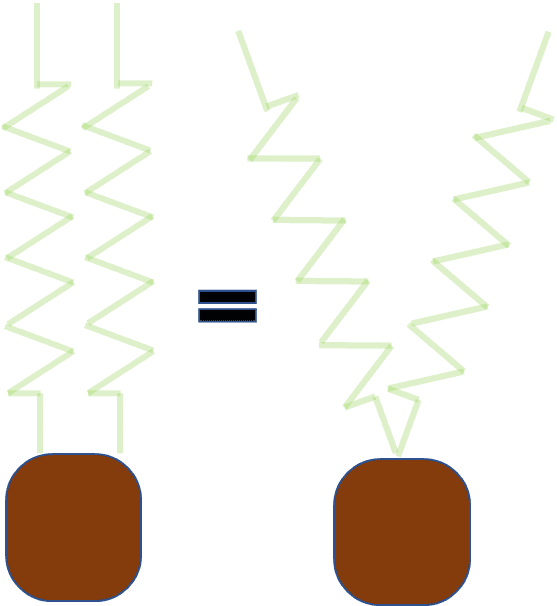I think I figured it out how these two can be equivalent in terms of work. Namely, they both store energy in stretching a distance ## x - l_o## of:

## U_{s} = k ( x - l_o )^2 ##

The figure on the left reduces to this by:

$$U_{left} = \frac{1}{2} k_{eff} ( x- l_o)^2 = \frac{1}{2} (2k)( x- l_o)^2 = k ( x - l_o )^2$$

The one on the right does this by the analysis in posts #7 and continuation #17

$$U_{right} = k ( x - l_o )^2$$

How could they end up doing the same amount of work? Distance. The force acting on the mass on right is reduced relative to the left, but the distance the force acts over is increased relative to the left. This is a result of the triangle inequality: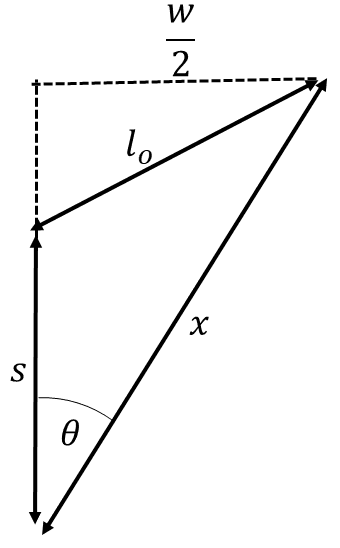$$s > x - l_o$$

So, I'm having more confidence for the result of the linear spring model result developed in #7 and #17.

As for how the experimental result ever agreed with the theory; There were two errors committed that cancel out to the linear spring model.

Error 1:
assuming constant acceleration which leads to the formula:

$$v^2 = 2 a d$$

Error 2:

Assuming constant acceleration we would arrive at:

$$a = \frac{2k d}{m}$$

Where ##d = x - l_o##.

However, we notice that only a single ##k## was used computationally (post #4 part (c)) in the model, namely saying ##a = \frac{k d}{m}##

Implying (either by hook or by crook):

$$v = d \sqrt{ \frac{2k}{m}}$$

These errors cancel to give the linear spring model using COE:

$$\frac{1}{2}mv^2 = k d^2 \implies v = d \sqrt{ \frac{2k}{m}}$$

That seems to explain how things experimentally agreed with "theory" IMOP ?

Last edited:
•I would like to see what the experimental relationship between the force F and and the axial displacement s actually is.
Thanks, Chestermiller. To start with the above remark: Here is the student's work (remember, we are talking about a 13-year old, so don't expect CERN-level standards):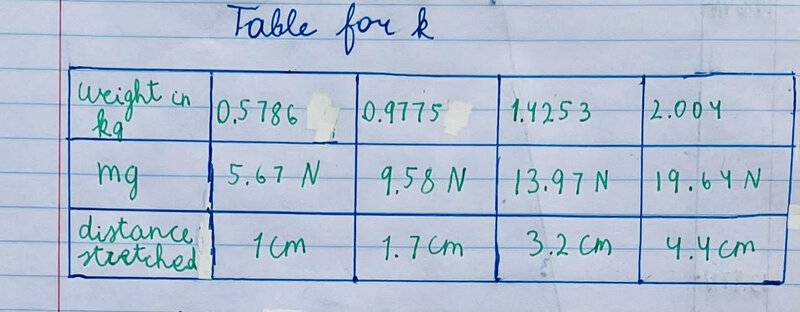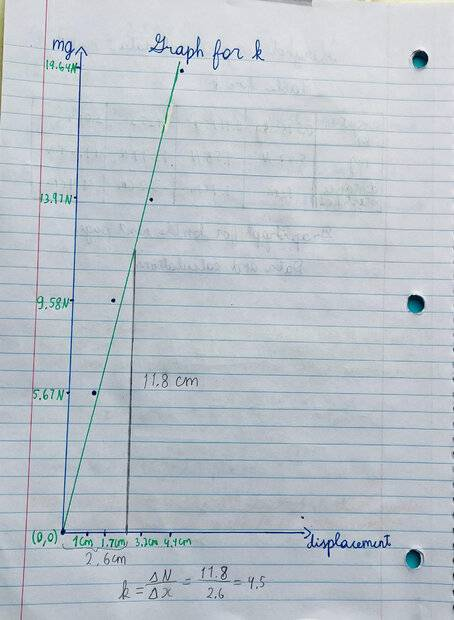Thanks, erobz. Super. By the way, for some reason in your post the last few LaTex strings didn't transform; they are decipherable, but do you think you could edit them so that they come out nicely as in the first part of the post?
Where .

However, we notice that only a single was used computationally (post #4 part (c)) in the model, namely saying ##a = \frac{k d}{m}

v = d \sqrt{ \frac{2k}{m}}

\frac{1}{2}mv^2 = k d^2 \implies v = d \sqrt{ \frac{2k}{m}}$$That seems to explain how things experimentally agreed with "theory" IMOP ? Thanks, erobz. Super. By the way, for some reason in your post the last few LaTex strings didn't transform; they are decipherable, but do you think you could edit them so that they come out nicely as in the first part of the post? Yeah, I saw that error. I probably edited it while you were making the post. They should be showing fine now? •nomadreid They should be showing fine now? Yes, thank you very much! The idea that the odd differences in experimental results, including getting the right results with the wrong theory in one case, and wrong results with the same theory in another, lies in the details of the geometric arrangements as you have outlined them seems eminently reasonable. •erobz Yes, thank you very much! The idea that the odd differences in experimental results, including getting the right results with the wrong theory in one case, and wrong results with the same theory in another, lies in the details of the geometric arrangements as you have outlined them seems eminently reasonable. Any chance we can get a picture of the student's actual slingshot? •nomadreid Any chance we can get an actual picture of the student's actual slingshot? I will write to her and ask her to send a picture; I will post it when I get it (or the morning after, if it comes while I am asleep). •erobz By integrating the actual data up to a displacement of 3 cm, I get a stored elastic energy of 0.231 J and a launch velocity of 4.45 m/s. •nomadreid I will add to the discussion my own experience when I tried to develop an experiment similar to the one described here. I didn't get very far and eventually I abandoned the idea primarily because the rubber bands I tried could not be trusted to provide the same force for the same displacement reproducibly. The cause for this lack of reproducibility was hysteresis. The force that the stretched band exerted depended on what had been done to it prior to the stretching. A simple experiment to investigate this was a "round-trip stretching" as opposed to the "one-way" measurement described here. I suspended a series of weights of increasing magnitudes and measured the displacement then reversed the order, reducing the hanging weights and remeasuring the distances. I found that the displacements for a given weight on the way up were lower than those on the way down. Furthermore, the results from the first round-trip set were not reproduced when I repeated with a second set using the same weights and procedure. I quit when I found out that the results also varied depending on how long I waited before starting the "return" trip with the largest mass stretching the band all the while. My point here is not to criticize this experiment but to offer a word of caution. Know thy band before comparing experimental results against an analysis that is based on Hooke's Law with a reproducible constant ##k##. •nomadreid and erobz Any chance we can get a picture of the student's actual slingshot?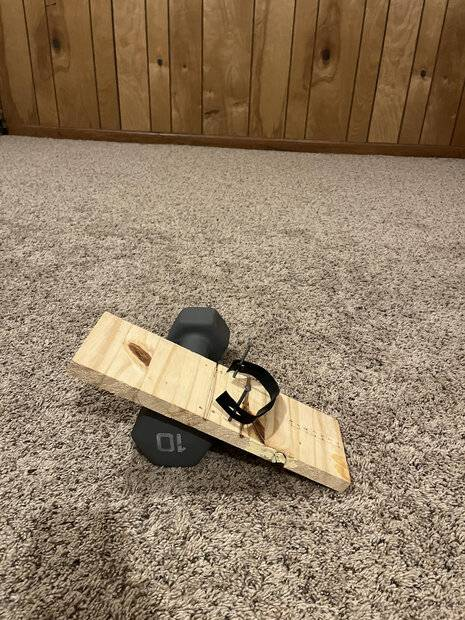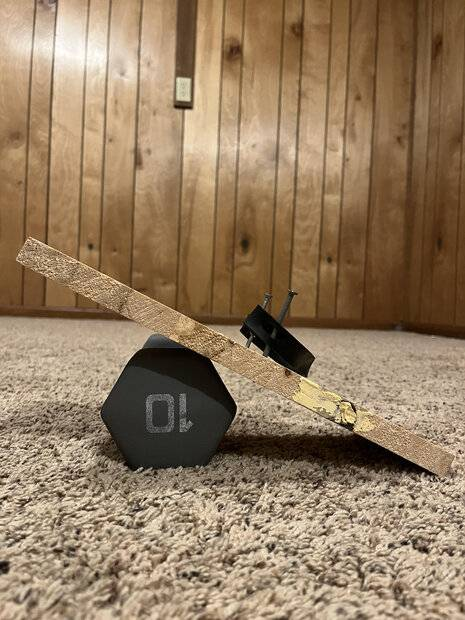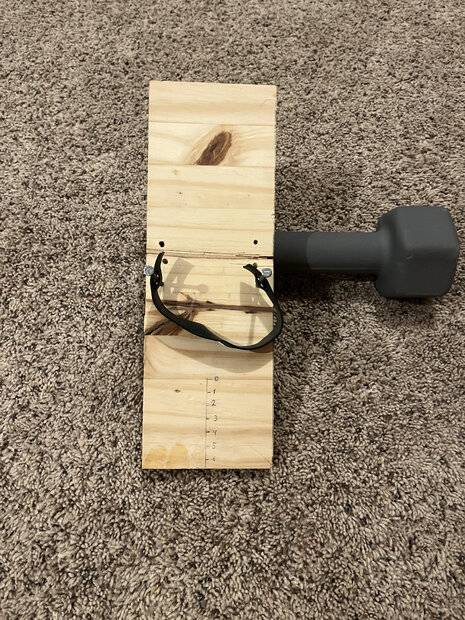By integrating the actual data up to a displacement of 3 cm, I get a stored elastic energy of 0.231 J and a launch velocity of 4.45 m/s. The horizontal distance that the projectile went was 116 cm when fired at an angle of 26 degrees to the horizontal. If I have the formula right(?) that the range = (v*sin2A)/g, this works backwards to 14 m/s. Am I doing something wrong here? I will add to the discussion my own experience when I tried to develop an experiment similar to the one described here. I didn't get very far and eventually I abandoned the idea primarily because the rubber bands I tried could not be trusted to provide the same force for the same displacement reproducibly. The cause for this lack of reproducibility was hysteresis. The force that the stretched band exerted depended on what had been done to it prior to the stretching. A simple experiment to investigate this was a "round-trip stretching" as opposed to the "one-way" measurement described here. I suspended a series of weights of increasing magnitudes and measured the displacement then reversed the order, reducing the hanging weights and remeasuring the distances. I found that the displacements for a given weight on the way up were lower than those on the way down. Furthermore, the results from the first round-trip set were not reproduced when I repeated with a second set using the same weights and procedure. I quit when I found out that the results also varied depending on how long I waited before starting the "return" trip with the largest mass stretching the band all the while. My point here is not to criticize this experiment but to offer a word of caution. Know thy band before comparing experimental results against an analysis that is based on Hooke's Law with a reproducible constant ##k##. Thanks, kuruman. I shall have the student do some more tests on the rubber band. The horizontal distance that the projectile went was 116 cm when fired at an angle of 26 degrees to the horizontal. If I have the formula right(?) that the range = (v*sin2A)/g, this works backwards to 14 m/s. Am I doing something wrong here? You mean (v2*sin2A)/g. Also, it looks like at release the projectile is about 2.7cm above the ground, which should add about 5cm to the range. Feeding 111cm into the formula I get 3.7m/s. 14m/s is 50kph. •nomadreid, Chestermiller and MatinSAR Ok, so this is where the actual geometry of the slingshot could come into play. The scale on the launch pad indicates they are measuring the distance ##s##, when we need ## d = x-l_o## for our formula.Using Pythagorean theorem, that implies that:$$ d = x- l_o = \sqrt{ \left( s + \sqrt{l_o^2 - \frac{w^2}{4} } \right)^2 + \frac{w^2}{4} } - l_o 

We can see by using that particular metric (##s##) slingshot geometry dependence has been introduced.

In general by the triangle inequality, ##s## should overestimate ##d##.

Last edited:
•